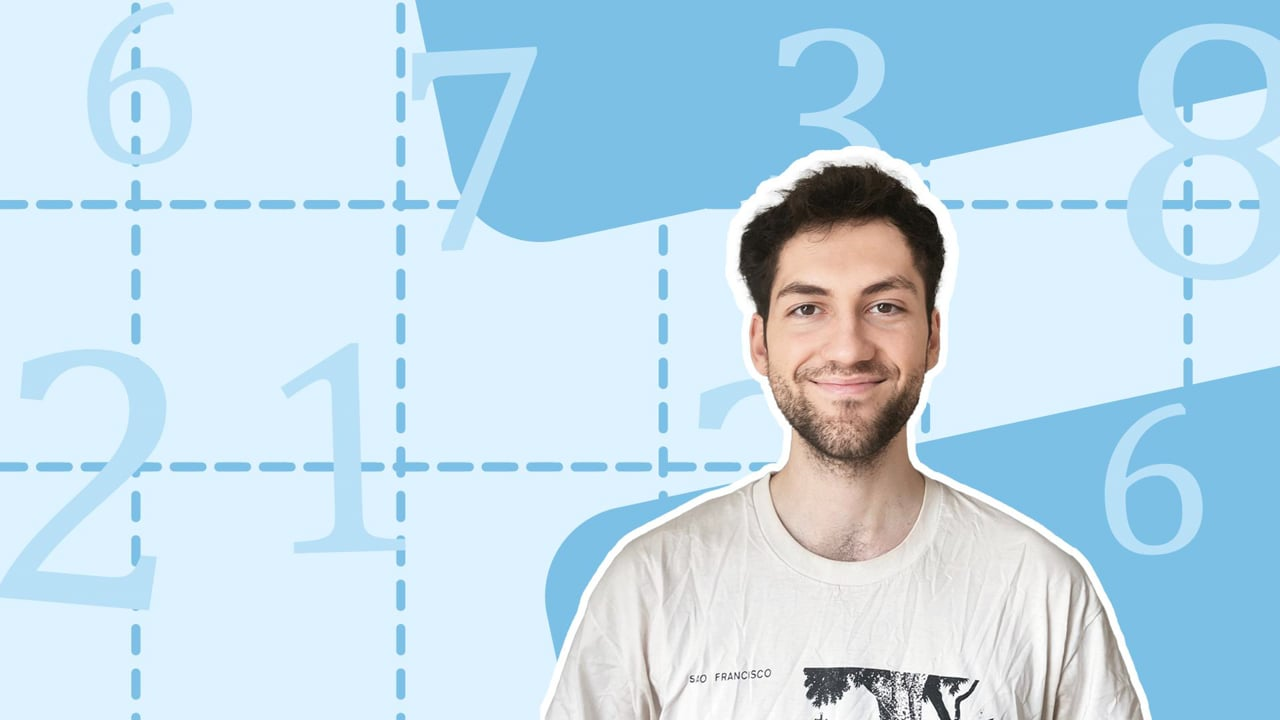Chapter OverviewMaths

Number and place value

Multiplication and division

Fractions

Measurement

Geometry - properties of shapes

Geometry - position and direction

Statistics

Ratio and proportion

Algebra

Maths

# Numbers to 10,000,0000%

Summary

# Numbers to 10,000,000

## ​​In a nutshell

Place value can be extended up to $10 \ 000 \ 000$ (and beyond).  To the left of the thousands column, the place value columns are ten thousands, hundred thousands, millions and ten millions. Reading, writing and comparing numbers up to $10 \ 000 \ 000$. follows a very similar process to smaller numbers.

## Reading and writing numbers to 10 000 000

When a number is written in digits, the position of the digit tells you its value. You can use place value tables to help you manage larger numbers.  Place value tables allow you to separate large numbers into their respective place value columns or rows, as seen below.​​

 Number ​$12 \ 345 \ 678$​​ ​$76 \ 482 \ 915$​​ Ten millions ​$1$​​ ​$7$​​ Millions ​$2$​​ ​$6$​​ Hundred thousands ​$3$​​ ​$4$​​ Ten thousands ​$4$​​ ​$8$​​ Thousands ​$5$​​ ​$2$​​ Hundreds ​$6$​​ ​$9$​​ Tens ​$7$​​ ​$1$​​ Ones ​$8$​​ ​$5$​​

##### Example 1

Write the number $12\ 345\ 678$ in words.

Fill in the digits in a place value table, as has been written above.

Combine the millions together to get

twelve million

Then, combine the thousands together to get

three hundred and forty five thousand

Finally, combine the hundreds, tens and ones together to get

six hundred and seventy-eight

Altogether, this can be written as

Twelve hundred, three hundred and forty five thousand, six hundred and seventy-eight.

## Comparing and ordering numbers up to 10 000 000

To compare two numbers, write them both in a place value chart and compare the largest digit to see which number is bigger. If the digits in the same place value row are the same, move to the next place value row. Continue this process until you reach your answer.

##### Example 2

Order the numbers $63\ 961\ 753$$52\ 852\ 862$ and $41\ 743\ 941$ from the largest to smallest.

Place these numbers into a place value table:

 Number ​$63 \ 961 \ 753$​​ ​$52 \ 852 \ 862$​​ ​$41 \ 743 \ 941$​​ Ten millions ​$6$​​ ​$5$​​ ​$4$​​ Millions ​$3$​​ ​$2$​​ ​$1$​​ Hundred thousands ​$9$​​ ​$8$​​ ​$7$​​ Ten thousands ​$6$​​ ​$5$​​ ​$4$​​ Thousands ​$1$​​ ​$2$​​ ​$3$​​ Hundreds ​$7$​​ ​$8$​​ ​$9$​​ Tens ​$5$​​ ​$6$​​ ​$4$​​ Ones ​$3$​​ ​$2$​​ ​$1$​​

Compare the digit in the ten millions row to order the numbers.  In this example, the digits in the ten millions row are all different, which makes it simple for you to order the numbers.

The number $\underline{63 \ 961 \ 753}$ is the largest, followed by $\underline{52 \ 852 \ 862}$ then $\underline{41 \ 743 \ 941}$.

## Want to find out more? Check out these other lessons!

Numbers to 1000

Numbers higher than 1000

Numbers to 10,000,000

Multiplying a 4-digit number by a 1 or 2-digit number

Dividing numbers up to 4 digits by a 1-digit number

Dividing numbers up to 4 digits by a 2-digit number

FAQs

• Question: How can you compare large numbers?

Answer: Place value charts can be used to compare numbers. Compare the digits in the column with the largest digits to see which number is greater than the others.

• Question: How can you read and write numbers up to 10 000 000?

Answer: You combine the millions together, then the thousands and finally the hundreds, tens and ones. Millions can be read out in a group, as can the thousands.

• Question: How can you compare numbers greater than one million?

Answer: To compare two numbers, write them both in a place value chart and compare the largest digit to see which number is bigger. If the digits in the same place value column are the same, move to the next place value column to the right. Continue this process until you reach your answer.​

Theory

Exercises